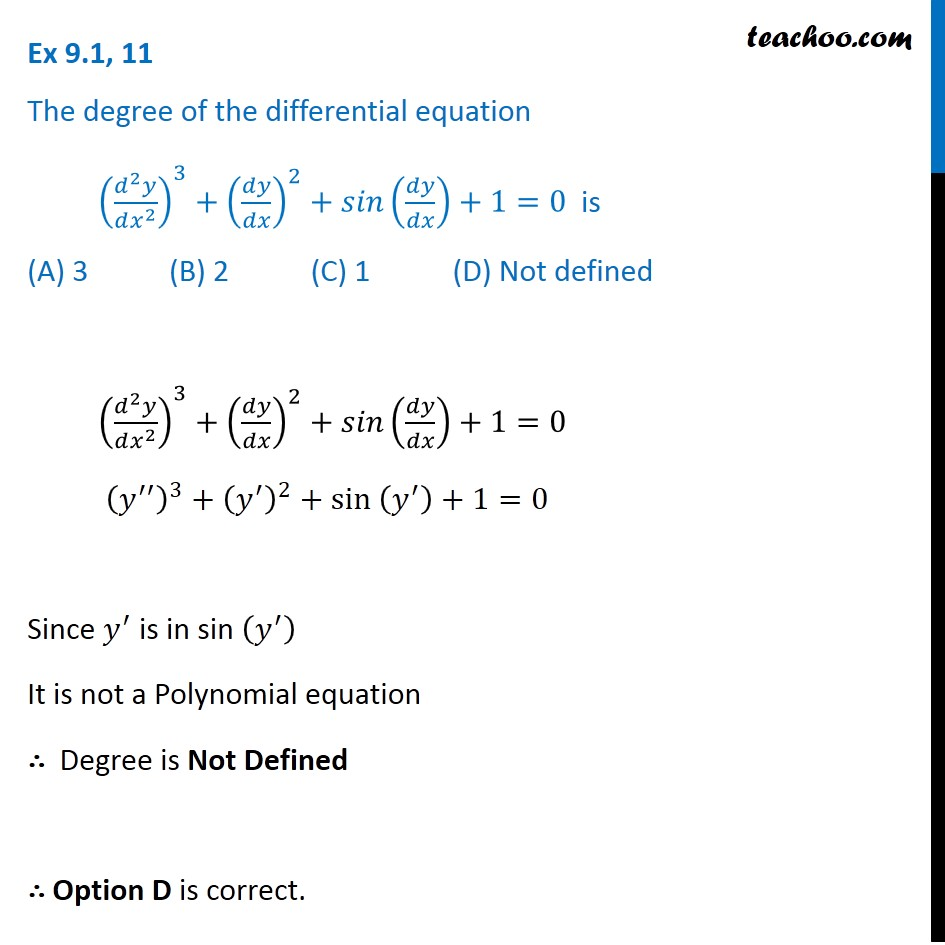Order and Degree

Chapter 9 Class 12 Differential Equations
Concept wiseIntroducing your new favourite teacher - Teachoo Black, at only ₹83 per month

### Transcript

Ex 9.1, 11 The degree of the differential equation ((𝑑^2 𝑦)/(𝑑𝑥^2 ))^3+(𝑑𝑦/𝑑𝑥)^2+𝑠𝑖𝑛(𝑑𝑦/𝑑𝑥)+1=0 is (A) 3 (B) 2 (C) 1 (D) Not defined ((𝑑^2 𝑦)/(𝑑𝑥^2 ))^3+(𝑑𝑦/𝑑𝑥)^2+𝑠𝑖𝑛(𝑑𝑦/𝑑𝑥)+1=0 (𝑦^′′ )^3+(𝑦^′ )^2+sin (𝑦^′ )+1=0 Since 𝑦^′ is in sin (𝑦^′ ) It is not a Polynomial equation ∴ Degree is Not Defined ∴ Option D is correct.